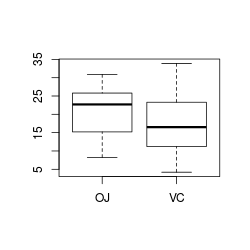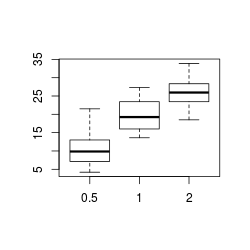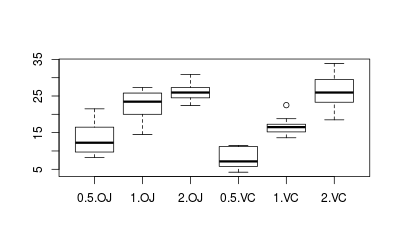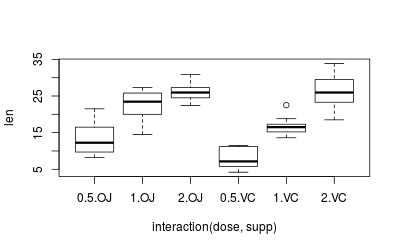## Problem

You want to make a box plot.

## Solution

This page shows how to make quick, simple box plots with base graphics. For more sophisticated ones, see Plotting distributions (ggplot2).

### Sample data

The examples here will use the `ToothGrowth` data set, which has two independent variables, and one dependent variable.

``````head(ToothGrowth)
#>    len supp dose
#> 1  4.2   VC  0.5
#> 2 11.5   VC  0.5
#> 3  7.3   VC  0.5
#> 4  5.8   VC  0.5
#> 5  6.4   VC  0.5
#> 6 10.0   VC  0.5
``````

Simple box plots of `len` against `supp` and `dose`:

``````boxplot(len ~ supp, data=ToothGrowth)

# Even though `dose` is a numeric variable, `boxplot` will convert it to a factor
boxplot(len ~ dose, data=ToothGrowth)
``````A boxplot of `len` against `supp` and `dose` together.

``````boxplot(len ~ interaction(dose,supp), data=ToothGrowth)
``````Note that `boxplot` and `plot` have much the same output, except that `plot` puts in axis labels, and doesn’t automatically convert numeric variables to factors, as was done with `dose` above.

``````plot(len ~ interaction(dose,supp), data=ToothGrowth)
``````